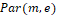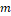### Relativistic Completion of Schrodinger’s Quantum Mechanics by the Ether Theory

•  David Zareski

#### Abstract

One recalls that we have shown in our precedent publications that the ether is an elastic isotropic medium. One presents the exact equation and its non-relativistic approximation that govern the ether in presence of a Schwarzschild-Coulomb field to which is submitted a Par(m,e)(particle of mass mand of electric charge e). We present the exact relativistic solution of this exact equation in the circular case. We prove that the Schrödinger equation is such a non-relativistic approximation, that is, is a particular case of the ether elasticity theory.

One recalls that the Schrödinger equation was obtained by the use of operators and not from the theory of elasticity. It follows that this manner of obtaining this equation from operators is arbitrary and does not permit to obtain its complete relativistic form, but permits to reach absurd conclusions as, e.g., the cat that, at the same moment, is alive and dead. One shows then that other results ensuing from the Schrödinger equation are particular cases of the non-relativistic equation that governs the elastic ether, like for example: the Bohr-Sommerfeld condition, and the eigenstates function equation.This work is licensed under a Creative Commons Attribution 4.0 License.
• ISSN(Print): 1916-9639
• ISSN(Online): 1916-9647
• Started: 2009
• Frequency: semiannual